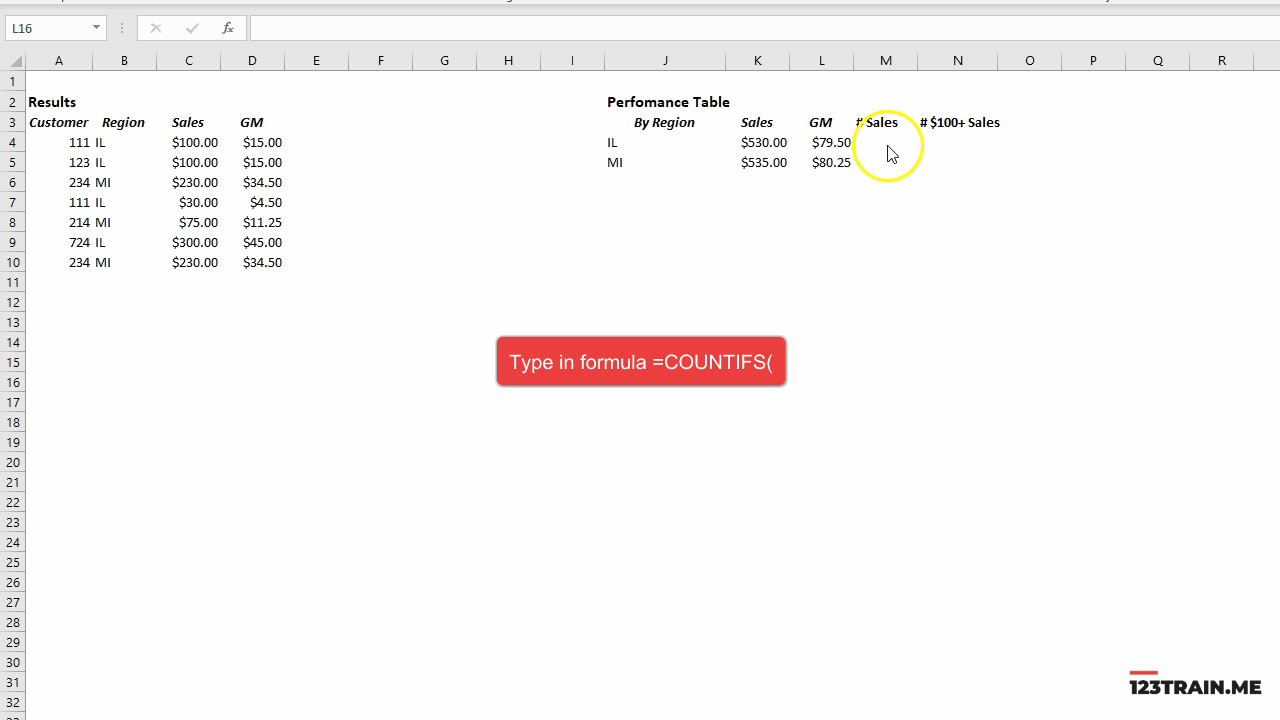top of page
Search

# COUNTIFSCOUNTIFS counts instances of all arguments being met.

COUNTIFS has the following arguments:

criteria_range:

A table range of values which will be compared against criteria.

criteria:

A value that must be met for an instance to be counted.

In the example above, we are trying to count number of sales in IL. To do that, we input column B (B:B) where we list states where a sale has happened. The second argument is our target argument. We are selecting IL (J4). The formula will count the number of rows value IL appears in the column B; which is 4 times.

In the cell N4 we want to know how many sales in IL were over \$100. There are two separate targets, one for condition with a state to be met and the other a condition of monetary value. These values reside in separate columns. To solve, we repeat exact argument we use to count number of IL instances for IL. Then, we add a second argument. The second argument selects column C (C:C) where the sales value is recorded. For criteria2, we write an expression >= which means greater or equal to. The equality sign must be in quotation marks and a value we compare against must be attached using & sign. Thus, our expression becomes ">="&100. Alternatively, we could store 100 as a value in a cell and give a cell reference. The formula will now look for matches in two different columns and return number of rows where conditions are true for both arguments; which is 3 rows.

Most common use of COUNTIFS function is matching values in columns and return number of rows which are true. It is possible to use the formula looking up values in rows and counting number of columns where conditions are true. To do that, for criteria_range we would give a range of one row and many columns.

For example:The highlighted cell above uses formula =COUNTIFS(4:4,"IL"). For the first argument, instead of a column, we gave a range of a row. For criteria, instead of cell reference, we gave a direct target IL. Quotation marks are needed to let Excel know we are looking for a text value. The return value is 2 because there are two columns where IL appears on row 4.

10 views

See All
bottom of page